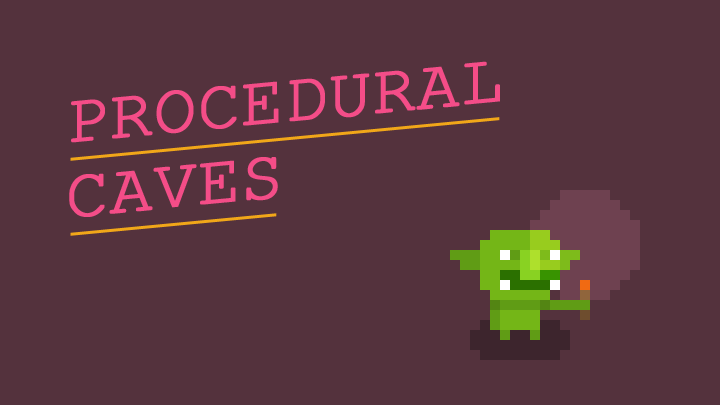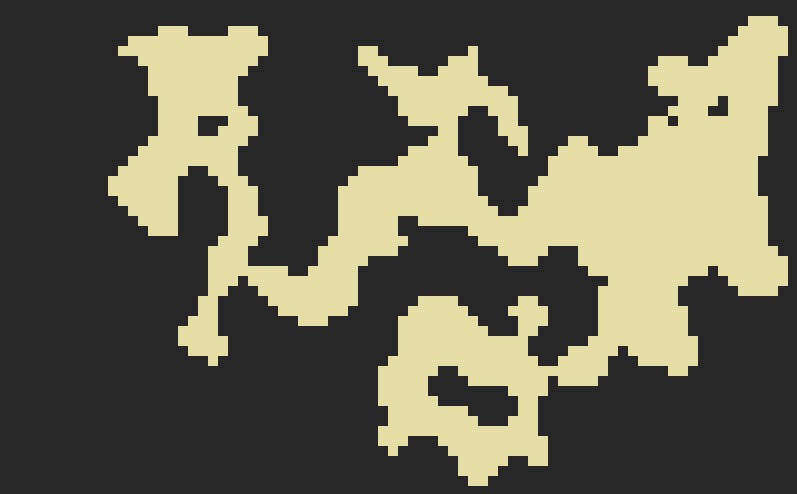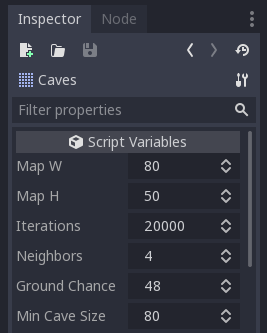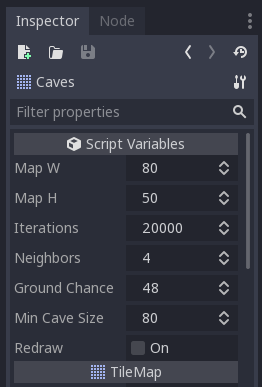# Procedural generation with Godot: Creating caves with Cellular AutomataWant to make a procedurally generated level for your game that includes caves or forests?  We did too, and here's how we learned to do it using Godot!

First we looked to see what info was already available; and while there is a lot of great resources like RogueBasin, few of them covered Godot or GDscript.

Fortunately, GDscript is very similar to Python, and we found a nice script on github where many procgen methods were created in Python.  So here we will show how we re-created the cellular automata one in Godot.  How's it look?sample cave generation using cellular automata

### Getting Started

To follow along and see how this works you can download the project files from my github repo.  The project is for Godot 3.1, and is just 1 Caves.tscn scene and about 200 lines of code.

To begin you want to create a scene in Godot and add a TileMap node to it, and then create a few tiles (you need ones for the ground and roof at least).  Next you want to attach a script to the TileMap node.

As a first step, lets add some variables that will let us tweak the procedural generation.  Paste the following into your script you added to the TileMap:

``````extends TileMap

export(int)   var map_w         = 80
export(int)   var map_h         = 50
export(int)   var iterations    = 20000
export(int)   var neighbors     = 4
export(int)   var ground_chance = 48
export(int)   var min_cave_size = 80

enum Tiles { GROUND, TREE, WATER, ROOF }
var caves = []``````

Once you save your script, if you select your TileMap node in the editor, you will now see some Script variables show up:These variables show up in the editor because we used the export keyword, and will allow us to tweak how our caves are generated.  So let's move on to the generation code.

### Generation Code

``````func generate():
clear()
fill_roof()
random_ground()
dig_caves()
get_caves()
connect_caves()``````

To generate our caves we first want to make a function describing all the tasks we need to do to create our caves.  The first task, clear(), is a method of the TileMap node and simply clears it of any existing tiles.  The rest of the tasks we need to create.

``````func fill_roof():
for x in range(0, map_w):
for y in range(0, map_h):
set_cell(x, y, Tiles.ROOF)``````

First, we loop through all the cells we will use in our TileMap, defined by the map_w and map_h variables, and set them to be roof tiles.

``````func random_ground():
for x in range(1, map_w-1):
for y in range(1, map_h-1):
if Util.chance(ground_chance):
set_cell(x, y, Tiles.GROUND)``````

Then, we want to loop through our TileMap again, and this time randomly change some of the roof tiles to ground tiles.  The chance this happens is defined by our ground_chance variable.  To make this work, we also have a Util.chance() method that we need to create.

Create a new script and call it Util.gd, and paste in the following code:

``````extends Node

# the percent chance something happens
func chance(num):
randomize()

if randi() % 100 <= num:  return true
else:                     return false

# Util.choose(["one", "two"])   returns one or two
func choose(choices):
randomize()

var rand_index = randi() % choices.size()
return choices[rand_index]``````

Now we want to make this Util.rb class available to all of our scenes, so we can use it in our Caves.tscn scene.  To do that we need to make it AutoLoad, by going to the Project menu -> Project Settings -> AutoLoad and adding Util.gd there.

Next up, let's create our actual caves:

``````func dig_caves():
randomize()
for i in range(iterations):
# Pick a random point with a 1-tile buffer within the map
var x = floor(rand_range(1, map_w-1))
var y = floor(rand_range(1, map_h-1))

# if nearby cells > neighbors, make it a roof tile
if check_nearby(x,y) > neighbors:
set_cell(x, y, Tiles.ROOF)

# or make it the ground tile
elif check_nearby(x,y) < neighbors:
set_cell(x, y, Tiles.GROUND)

func check_nearby(x, y):
var count = 0
if get_cell(x, y-1)   == Tiles.ROOF:  count += 1
if get_cell(x, y+1)   == Tiles.ROOF:  count += 1
if get_cell(x-1, y)   == Tiles.ROOF:  count += 1
if get_cell(x+1, y)   == Tiles.ROOF:  count += 1
if get_cell(x+1, y+1) == Tiles.ROOF:  count += 1
if get_cell(x+1, y-1) == Tiles.ROOF:  count += 1
if get_cell(x-1, y+1) == Tiles.ROOF:  count += 1
if get_cell(x-1, y-1) == Tiles.ROOF:  count += 1
return count``````

Here we are choosing a random cell in our TileMap, and then checking the surrounding cells to see if they are ground or roof tiles, then updating it to also be a ground or roof tile based off how many neighbors.  We do this how ever many times you specify in the iterations variable.  When done many times (20,000 in this demo), it carves out our caves for us.

### Connecting Caves

With the above code in place, you have actual caves you can generate.  But there is a problem, the caves are not connected, which makes for a rather unplayable map.Before we can connect the caves, we need to get the caves first and store them in a list.  To do this we use a flood fill.  This works like the paint bucket tool in drawing apps - you pick a ground cell and remove it (changing it temporarily to a roof), then check its surrounding cells; if they are also ground remove them, and check their surrounding cells, until no ground cells are found - then store all those cells as a cave, and start again until you have mapped all caves.

``````func get_caves():
caves = []

for x in range (0, map_w):
for y in range (0, map_h):
if get_cell(x, y) == Tiles.GROUND:
flood_fill(x,y)

for cave in caves:
for tile in cave:
set_cellv(tile, Tiles.GROUND)

func flood_fill(tilex, tiley):
var cave = []
var to_fill = [Vector2(tilex, tiley)]
while to_fill:
var tile = to_fill.pop_back()

if !cave.has(tile):
cave.append(tile)
set_cellv(tile, Tiles.ROOF)

var north = Vector2(tile.x, tile.y-1)
var south = Vector2(tile.x, tile.y+1)
var east  = Vector2(tile.x+1, tile.y)
var west  = Vector2(tile.x-1, tile.y)

for dir in [north,south,east,west]:
if get_cellv(dir) == Tiles.GROUND:
if !to_fill.has(dir) and !cave.has(dir):
to_fill.append(dir)

if cave.size() >= min_cave_size:
caves.append(cave)``````

With our caves stored, we only have one step left, and that is to connect them.  To do this we want to loop through our caves, picking a point in each, and then walk a path between them.  To make the path feel more natural then a straight line, we will do a random/drunken walk, weighing the directions to guide us.

``````func connect_caves():
var prev_cave = null
var tunnel_caves = caves.duplicate()

for cave in tunnel_caves:
if prev_cave:
var new_point  = Util.choose(cave)
var prev_point = Util.choose(prev_cave)

# ensure not the same point
if new_point != prev_point:
create_tunnel(new_point, prev_point, cave)

prev_cave = cave

# do a drunken walk from point1 to point2
func create_tunnel(point1, point2, cave):
randomize()          # for randf
var max_steps = 500  # so editor won't hang if walk fails
var steps = 0
var drunk_x = point2
var drunk_y = point2

while steps < max_steps and !cave.has(Vector2(drunk_x, drunk_y)):
steps += 1

# set initial dir weights
var n       = 1.0
var s       = 1.0
var e       = 1.0
var w       = 1.0
var weight  = 1

# weight the random walk against edges
if drunk_x < point1.x: # drunkard is left of point1
e += weight
elif drunk_x > point1.x: # drunkard is right of point1
w += weight
if drunk_y < point1.y: # drunkard is above point1
s += weight
elif drunk_y > point1.y: # drunkard is below point1
n += weight

# normalize probabilities so they form a range from 0 to 1
var total = n + s + e + w
n /= total
s /= total
e /= total
w /= total

var dx
var dy

# choose the direction
var choice = randf()

if 0 <= choice and choice < n:
dx = 0
dy = -1
elif n <= choice and choice < (n+s):
dx = 0
dy = 1
elif (n+s) <= choice and choice < (n+s+e):
dx = 1
dy = 0
else:
dx = -1
dy = 0

# ensure not to walk past edge of map
if (2 < drunk_x + dx and drunk_x + dx < map_w-2) and \
(2 < drunk_y + dy and drunk_y + dy < map_h-2):
drunk_x += dx
drunk_y += dy
if get_cell(drunk_x, drunk_y) == Tiles.ROOF:
set_cell(drunk_x, drunk_y, Tiles.GROUND)

# optional: make tunnel wider
set_cell(drunk_x+1, drunk_y, Tiles.GROUND)
set_cell(drunk_x+1, drunk_y+1, Tiles.GROUND)``````

And that's it!  You now have procedural caves generated in Godot that are connected to each other.

### Bonus: Godot Tools

One really cool feature of Godot is that its easy to make a script run inside the editor.  To do this, we add the tool keyword to the beginning of the script.  In our case, we would want to add it to both the TileMap script, and to the Util.gd script.

``````tool
extends TileMap

export(bool)  var redraw  setget redraw

func redraw(value = null):

# only do this if we are working in the editor
if !Engine.is_editor_hint(): return

generate()``````

With tool added, we can make a new checkbox exported variable called Redraw, and when we click it, it will call the generate() function to create our caves.  Now we can tweak our procedural variables right inside the editor, and click redraw to see the results.A cool use for this is it turns Godot into a level editor for you, allowing you to procedurally generate levels, save them as scenes, and then further customize them in the editor, tweaking the tiles, adding enemies and objects, etc.

### That's a Wrap!

We hope you enjoyed our first tutorial on Godot.  If you want to get notified of our new posts or simply chat with us follow @abitawake on Twitter =)